10 篇文章 0 订阅

一. 常见的特征类型二. 编码

2.1 序号编码（Ordinal Encoding）

sklearn.preprocessing中的OrdinalEncoder进行处理，可以通过两种方式进行编码：

• 方法1：先将数据categorical_df载入encoder中，再将载入的数据进行OrdinalEncoder编码
• 方法2: 直接载入encoder并编码
import pandas as pd
from sklearn.preprocessing import OrdinalEncoder

categorical_df = pd.DataFrame({'my_id': ['101', '102', '103', '104'],
'name': ['allen', 'bob', 'chartten', 'dory'],
'place': ['third', 'second', 'first', 'second']})
print(categorical_df)
print('--'*20)

encoder = OrdinalEncoder() #创建OrdinalEncoder对象

# 方法1
encoder.fit(categorical_df) #将数据categorical_df载入encoder中
categorical_df = encoder.transform(categorical_df) #将载入的数据进行OrdinalEncoder

# 方法2
categorical_df = encoder.fit_transform(categorical_df) #代替上面的fit()和transform(),一步到位

print(categorical_df)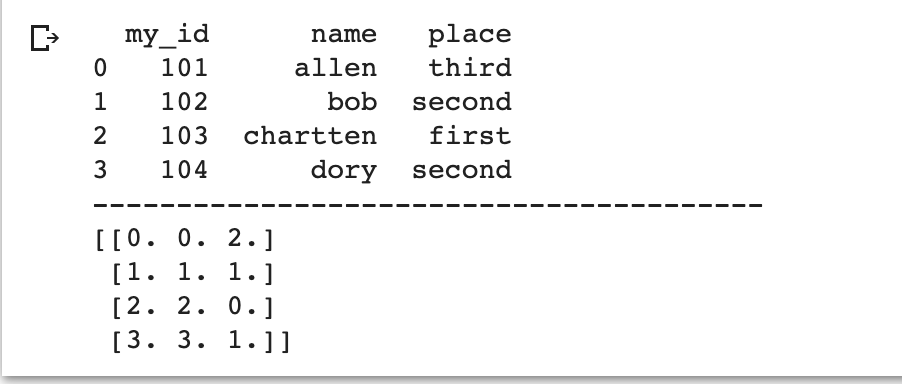import pandas as pd
from sklearn.preprocessing import OrdinalEncoder

categorical_df = pd.DataFrame({'my_id': ['101', '102', '103', '104'],
'name': ['allen', 'bob', 'chartten', 'dory'],
'place': ['third', 'second', 'first', 'second']})

# In the 'name' column, I want 'dory' to be 0, 'bob' to be 1, 'chartten' to be 2, and 'allen' to be 3
# In the 'place' column, I want 'first' to be 0, 'second' to be 1, and 'third' to be 2
name_categories = ['dory', 'bob', 'chartten', 'allen']
place_categories = ['first', 'second', 'third']

encoder = OrdinalEncoder(categories=[name_categories, place_categories])

categorical_df[['name', 'place']]= encoder.fit_transform(categorical_df[['name', 'place']])

categorical_df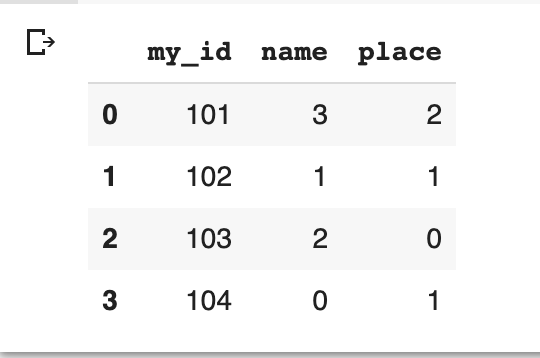2.2独热编码（One-hot Encoding）

import pandas as pd
from sklearn.preprocessing import OneHotEncoder

categorical_df = pd.DataFrame({'my_id': ['101', '102', '103', '104'],
'name': ['allen', 'bob', 'chartten', 'dory'],
'place': ['third', 'second', 'first', 'second']})
print(categorical_df)
print('--'*20)

encoder = OneHotEncoder()
encoder.fit_transform(categorical_df[['name']]).toarray()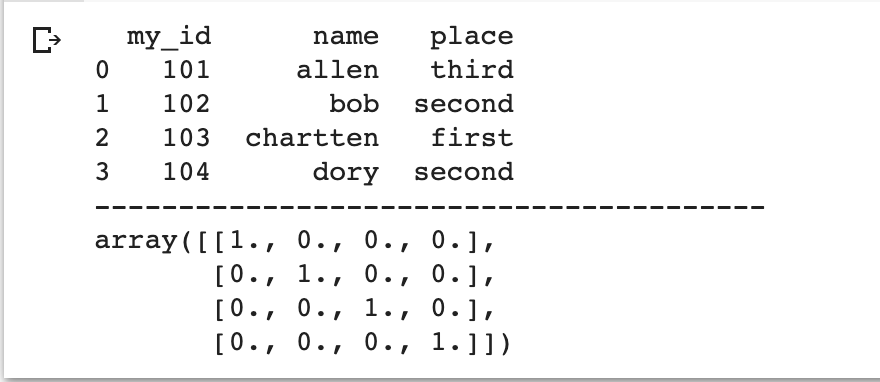import pandas as pd
from sklearn.preprocessing import OneHotEncoder

categorical_df = pd.DataFrame({'my_id': ['101', '102', '103', '104'],
'name': ['allen', 'bob', 'chartten', 'dory'],
'place': ['third', 'second', 'first', 'second']})
print(categorical_df)
print('--'*20)

encoder = OneHotEncoder(categories=[['dory', 'bob', 'chartten', 'allen']])
encoder.fit_transform(categorical_df[['name']]).toarray()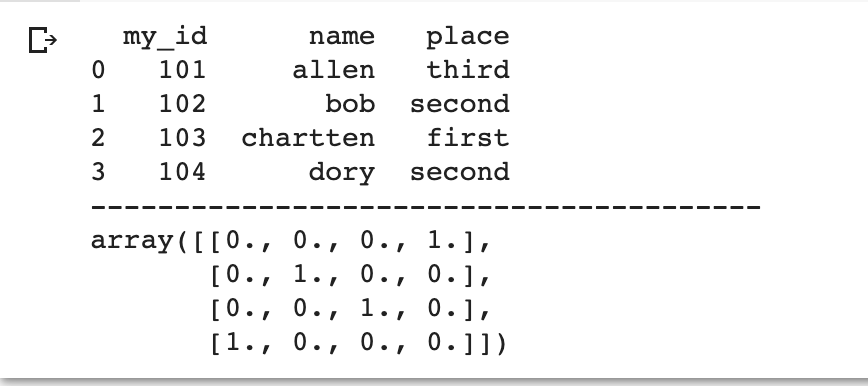2.3 标签编码 (Label Encoding)

Label Encoding是给某一列数据编码，而Ordinal Encoding是给所有的特征编码。因此Label Encoding常用于给标签(label)编码，而Ordinal Encoding常用于给数据集中的特征编码。

import pandas as pd
from sklearn.preprocessing import LabelEncoder

categorical_df = pd.DataFrame({'my_id': ['101', '102', '103', '104'],
'name': ['allen', 'bob', 'chartten', 'dory'],
'place': ['third', 'second', 'first', 'second'],
})
print(categorical_df)
print('--'*20)

encoder = LabelEncoder()
categorical_df['place']=encoder.fit_transform(categorical_df['place'])
categorical_df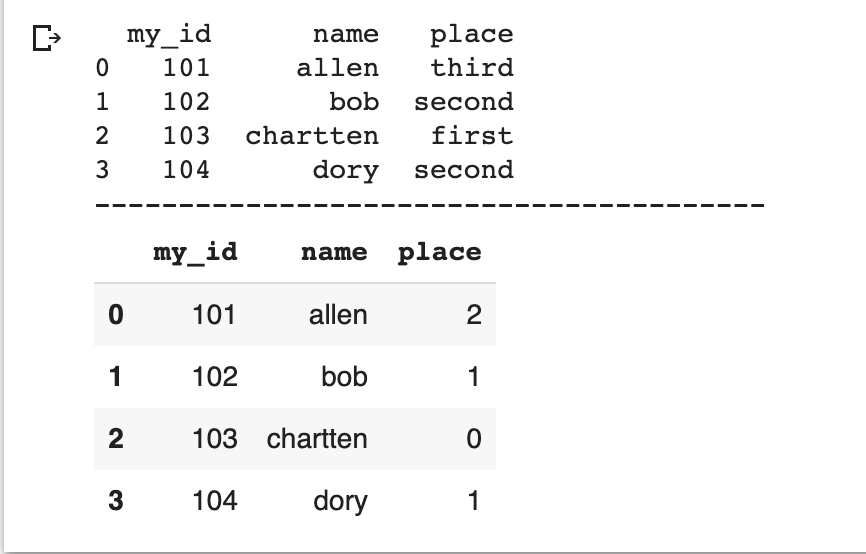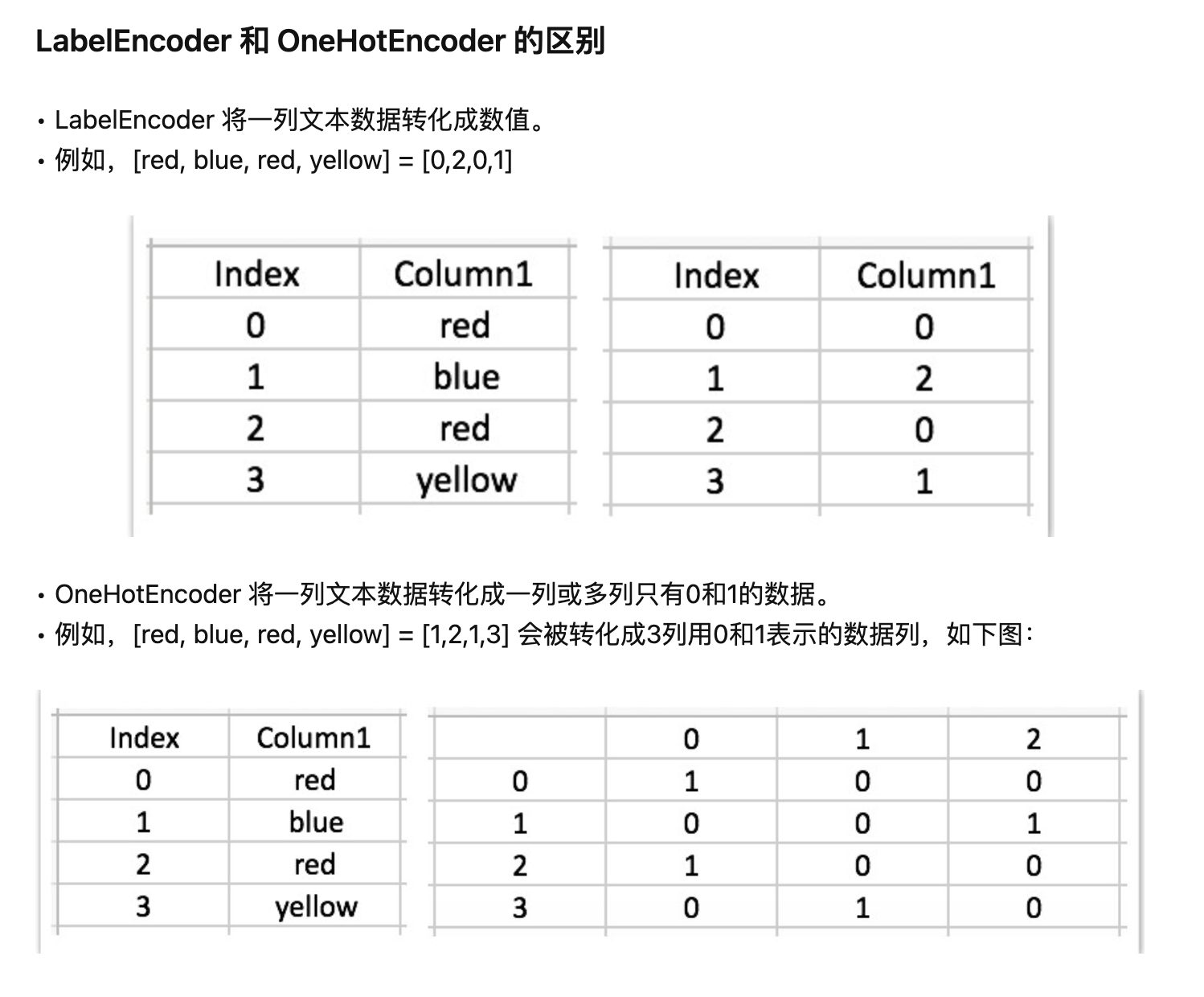• 特征数据类型
对于定类类型的数据，建议使用one-hot encoding。定类类型就是纯分类，不排序，没有逻辑关系。比如性别分男和女，男女不存在任何逻辑关系，我们不能说男就比女好，或者相反。再者，中国各省市分类也可以用独热编码，同样各省不存在逻辑关系，这时候使用one-hot encoding会合适些。但注意，一般会舍去一个变量，比如男的对立面肯定是女，那么女就是重复信息，所以保留其中一个变量即可。
对于定序类型的数据，建议使用label encoding。定序类型也是分类，但有排序逻辑关系，等级上高于定类。比如，学历分小学，初中，高中，本科，研究生，各个类别之间存在一定的逻辑，显然研究生学历是最高的，小学最低。这时候使用Label encoding会显得更合适，因为自定义的数字顺序可以不破坏原有逻辑，并与这个逻辑相对应。

• 所使用的模型
数值大小敏感的模型必须使用one-hot encoding。典型的例子就是LR和SVM。二者的损失函数对数值大小是敏感的，并且变量间的数值大小是有比较意义的。而Label encoding的数字编码没有数值大小的含义，只是一种排序，因此对于这些模型都使用one-hot encoding。
数值大小不敏感的模型（如树模型）不建议使用one-hot encoding。一般这类模型为树模型。如果分类类别特别多，那么one-hot encoding会分裂出很多特征变量。这时候，如果我们限制了树模型的深度而不能向下分裂的话，一些特征变量可能就因为模型无法继续分裂而被舍弃损失掉了。因此，此种情况下可以考虑使用Label encoding。

2.4 频数编码（Frequency Encoding/Count Encoding）

import category_encoders as ce
features = ['Peking', 'Peking', 'Shanghai', 'Peking', 'Guangzhou', 'Shanghai']
count_enc = ce.CountEncoder()
count_enc.fit_transform(features)

2.5 目标编码（Target Encoding）

01-21

01-301858
02-27727
09-251万+
09-151860
01-035118
10-211870
08-27101
03-041925
01-263万+
11-11904
10-317878
10-22108
09-242130
09-16181
06-01312
01-14485
01-116377
12-047174
09-2738366Kevin

¥2 ¥4 ¥6 ¥10 ¥20余额支付 (余额：-- )扫码支付获取中扫码支付点击重新获取扫码支付1.余额是钱包充值的虚拟货币，按照1:1的比例进行支付金额的抵扣。
2.余额无法直接购买下载，可以购买VIP、C币套餐、付费专栏及课程。余额充值